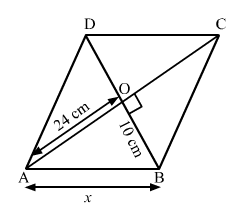# The area of a rhombus is 480 cm2, and one of its diagonals measures 48 cm.

Question:

The area of a rhombus is 480 cm2, and one of its diagonals measures 48 cm. Find

(i) the length of the other diagonal,

(ii) the length of each of its sides, and

(iii) its perimeter.

Solution:

It is given that,
Area of rhombus = 480 cm2.
One of the diagonal = 48 cm.

(i) Area of the rhombus $=\frac{1}{2} \times d_{1} \times d_{2}$

$\Rightarrow 480=\frac{1}{2} \times 48 \times d_{2}$

$\Rightarrow 480=24 \times d_{2}$

$\Rightarrow d_{2}=\frac{480}{24}$

$\Rightarrow d_{2}=20 \mathrm{~cm}$

$\Rightarrow 480=\frac{1}{2} \times 48 \times d_{2}$

$\Rightarrow 480=24 \times d_{2}$

$\Rightarrow d_{2}=\frac{480}{24}$

$\Rightarrow d_{2}=20 \mathrm{~cm}$

Hence, the length of the other diagonal is 20 cm.

(ii) We know that the diagonals of the rhombus bisect each other at right angles.In right angled ∆ABO,

$A B^{2}=A O^{2}+O B^{2}$        (Pythagoras Theorem)

$\Rightarrow A B^{2}=24^{2}+10^{2}$

$\Rightarrow A B^{2}=576+100$

$\Rightarrow A B^{2}=676$

$\Rightarrow A B=26 \mathrm{~cm}$

Hence, the length of each of the sides of the rhombus is 26 cm.

(iii) Perimeter of the rhombus = 4 × side

= 4 × 26

= 104 cm

Hence, the perimeter of the rhombus is 104 cm.